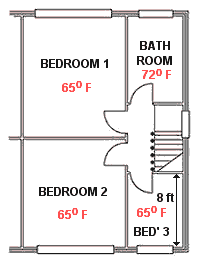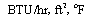# Example of heat loss calculation (imperial)

A simple example applied to a semi-detached property

The background to calculating heat loss from a property is covered on a separate page on this site, Before looking at this example, have a look at the page on sizing so you understand the basic principles.For this example, apart from the dimensions shown on the above drawings, it is also necessary to know:

1. All rooms are 8 ft in height.
2. All external walls are 11 inch cavity without insulation.
3. The party wall is 9 inch solid brick.
4. The internal walls are all plaster, 4.5 inch brick, plaster.
5. The floor is suspended timber.
6. All glazing is UVPC with double glazing.
7. The outside design temperature to 30° F.
8. The temperature in the adjoining property is not known, so assume a 5° F temperature difference.
9. The design temperatures for the room - look at this page.
10. The large windows are 10 ft x 4 ft, the smaller windows are 4 ft x 4 ft.
11. The roof is tiled on felt with 100 mm insulation.
12. The plan is not to scale !!

In this example we look in detail at a single room (the lounge).

1. Consider the 4 walls in turn and calculate the area of each type of fabric:
• Front wall:
1. Total wall 14 ft x 8 ft = 112 sq ft
2. Window 10 ft x 4 ft = 40 sq ft
3. So cavity wall - 112 - 40 = 72 sq ft
• Party wall:
1. Total wall area 15 ft x 8 ft = 120 sq ft
• Wall to Dining Room:
1. There is no temperature difference across this wall, so no flow of thermal energy so there is no need to calculate the area.
• Wall to Hall:
1. Total wall 15 ft x 8 ft = 120 sq ft
2. The door is treated as if it were wall
• Ceiling and floor areas:
1. 15 ft x 14 ft = 210 sq ft:
2. Using the figures above, the U values (look at this page) and the temperature difference across each wall/ceiling/floor the heat loss can be calculated (area x U value x temp difference).
 area (sq ft) U valuetemp difference total Front wall : cavity wall 72 0.18 40 518.4 Window 40 0.51 40 816 Party Wall 120 0.38 5 228 Dining Room wall - 0.39 0 0 Hall wall 120 0.39 10 468 Ceiling 210 0.29 5 304.5 Floor 210 0.12 40 1008 Total fabric loss = 3342.9

So the total heat loss through the fabric of the building is 3345 BTU
3. Now to calculate the heat loss due to air changes.
• volume of the room = 14 x 15 x 8 = 1,680 cubic ft
air change = 1 per hour (dependant upon room - look at this page)
so heat loss through air changes is
1,680 x 1 x 0.02 x 40 = 1344 BTU
4. Add the results from 2 and 3 together, gives the total heat loss of per hour of:
• 3345 + 1344 = 4689 BTU/hr

This is the calculations for the lounge, it is now necessary to carry out calculations for all the other rooms in the house. Note that where heat 'losses' occur across internal walls or floor/ceiling, one room will loss the heat while the other room will gain it. In the calculations, the room gaining the heat will show a negative heat loss for that particular part of the building fabric.

 fabric loss air change loss total (BTU/hr) Dining room 3391 3046 6437 Lounge 3343> 1344 4687 Kitchen 1714 941 2655 Hallway 1501 1250 2751 Bedroom 1 1162 666 1828 Bedroom 2 1678 588 2266 Bedroom 3 1009 134 1143 Bathroom 2192 1129 3321 Total for house = 25,088

The results of the calculations for all the room in the example house are shown to the right. This indicates the amount of heat required to be generated within each room to keep them at the design temperatures. Not only is this necessary to determine the appropriate size of the radiators, it is also required to size the pipes for water based central heating.

When all the values have been added together, the final figure is an indication of the boiler size required to heat the house (note: it does not take into account any additional heating required for the domestic hot water system).

Detailed workings of the calculations for the complete house are shown on another page on this site.

These simplistic calculations do not take into account any heat produced by the inhabitants or their activities (such as cooking, washing etc.). It can be changed by improving (i.e. reducing) the amount of air changes by increased draught excluding, improved insulation of the fabric or accepting a lower design temperature within any of the rooms.

Generally there is no point in trying to calculate the heat loss figures too accurately, its main purpose is to give an indication of the size of radiators and boiler required. Knowing these heat loss figures should ensure that the chosen radiators and boiler are not unnecessarily under-rated nor over-rated; some over-rating will be unavoidable as the final calculated figure will not absolutely match the rated output of any radiator or boiler.